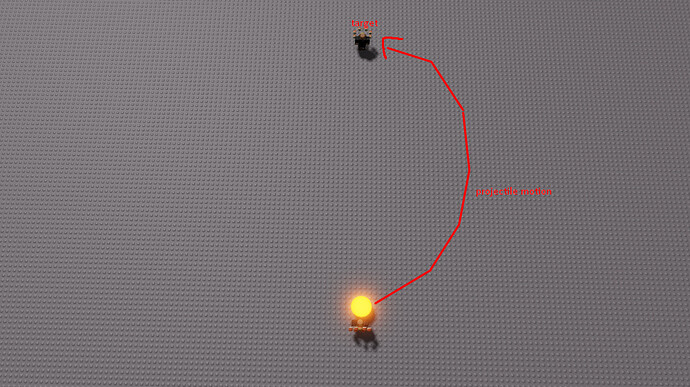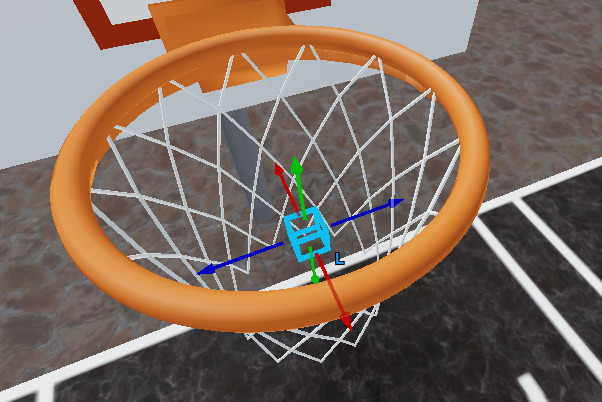# Modeling a projectile's motion

Never knew this was possible! I used to simulate it in a for loop then just put bricks at its positions

2 Likes

This topic can use integral calc, but it mostly regards with physics and projectile motion.
A short 13 minute video to help you understand a bit about projectile motion is rather simple in the way the video explains it. I’m obviously not going to type a whole page about projectile motion when there’s a video that does it all.
https://youtu.be/M8xCj2VPHas
This is for projectile motion, and you’ll immediately notice it’s very similar to the tutorial. In the equations, they just use different variables and the terms are in different order, but I’m sure you can easily understand the equations.

9 Likes

Love the tutorial, especially showing the importance of math to doing this stuff!

One question though, if I don’t have a sphere following the path but a rod, what would be the best way to rotate it so that it is always pointing in the direction of the arc? At first I was thinking about doing a BodyAngularVelocity from 0 to pi, but that won’t work in a local script. Would I have to use CFrames then?

EDIT: Ok, here’s what I’ve got so far:
Right before the `while (nt < t*2)` loop, I put this in to set the starting angle of the projectile and point it at the target.

``````local angle = math.pi / 4
p.CFrame = CFrame.new(p.Position, hrp.Position)``````

Then, inside of the while loop I have:

``p.CFrame = p.CFrame * CFrame.Angles(0, angle, 0)``

And after the RenderStepped update:

``angle = (math.pi / 4) - ((nt / (t * 2)) * (math.pi / 2))``

What this should do is start the projectile pointing at the target, then up at a 45 degree angle. Then, on each render step, it subtracts from that angle a proportion of the full rotation of the projectile. The end result is moving from a +45 angle to a -45 angle by the end of the animation.

However, when I run it, I don’t see the projectile actually rotating. I printed out all my angles as it calculates them, and they look correct, running from +.76 to -.80.

Can anyone see what I’m doing wrong?

EDIT 2: I figured out some of the mistakes I made in that code. Turns out that I can’t do the CFrame.Angles with the argument of the angle I want it pointed to, but I have to use an argument for how much I want it rotated by.

So instead of calculating the necessary angle for each step, I just have to calculate the change in angle between each frame:

``local angleDelta = ((math.pi / 4) / t) / 60``

Because I couldn’t see a good way to calculate the number of times the animation loop will run, I took the amount of the rotation (45 degrees) divided by the time to take that rotation (t) divided by the number of frames per second (I assumed 60 because that is the cap and I don’t know a good way to get the actual value).

Then, in the animation loop, I have:

``projectile.CFrame = projectile.CFrame * CFrame.Angles(-(angleDelta), 0, 0)``

Now when I have a static projectile that is not moving, it rotates the correct amount in the correct duration. But when I try to combine it with the CFrame change from `projectile.CFrame = CFrame.new((.5 * g * nt * nt) + (v0 * nt) + source.Position)`, it doesn’t rotate. I also tried combining the two together with a multiplication, but that doesn’t work either.

Any ideas why I can’t get the two working together?

3 Likes

Sorry I for whatever reason didn’t see this question when you originally posted it.

For this I would use the velocity equation to get the direction you’re object is going to be facing and then from there you can use `CFrame.lookAt` or `CFrame.fromMatrix`.

``````local g, v0, x0 -- define these

local function x(t)
return 0.5*g*t*t + v0*t + x0
end

local function v(t)
return g*t + v0
end

local t = 0
game:GetService("RunService").Heartbeat:Connect(function(dt)
t = t + dt
local position = x(t)
local velocity = v(t)

local cframe = CFrame.lookAt(position, position + velocity)
end)
``````

Now the one issue you may find with this is when the parabola reaches its peak then velocity will have a magnitude of zero (as shown when deriving the max jump height in the OP).

In that case we need to handle the situation a little differently:

``````local t = 0
game:GetService("RunService").Heartbeat:Connect(function(dt)
t = t + dt
local position = x(t)
local velocity = v(t)

if velocity:Dot(velocity) == 0 then -- magnitude^2 == 0
velocity = v(t - dt) * Vector3.new(1, 0, 1)
end

local cframe = CFrame.lookAt(position, position + velocity)
end)
``````

Hope that helps!

21 Likes

This is exactly what I was looking for! Thank you so much1 Like

Thank you so much for the resource!

It turns out that the trajectory will have inaccuracies for reasons other than collision-based velocity damping. One of them is the integration method Roblox is currently using (the formula that updates the object position based on its velocity, and its velocity based on its acceleration). Users @GFink, @GloriedRage and I have recently figured how to suppress this offset, if you’d like to take a look!

1 Like

I saw this thread and i wanted to ask you something. How can i make a projectile (or any part etc.) move into a special trajectory (not roblox world physics) like in Original Snido Life. I mean projectile goes to mouse point but not up and down but from left to right. Also, self growing trees from Shindo Life.4 Likes

Is it possible to somehow overcome the small delay before the projectile starts moving when velocity and not heartbeat is used. When i replicate the code here in a server script there is a small but very noticeable delay before the projectile starts moving and it really is quite infuriating. I am hoping there is some simple way to resolve this issue.

here for reference

1 Like

I have this exact same issue. I would go about maybe having a premade projectile (and then set the network ownership)? But, I would prefer not to do that in my game due to the fact that player’s can customize the tools they throw and I would just rather clone the handle. You could try implementing buffer module but I am unsure of how to do that.

i ended up implenting the module but i am not too pleased with it. Using pre made projectiles also didn’t help, nor did network ownership help. Honestly i am just disappointed with Roblox for this being an issue.

There will always be a slight delay when a client makes a request to the server. You should look into lag compensation so that you can minimize the visual impact.

(also, never have the server itself render your projectiles, do it locally)

Here are some resources to look at:

https://www.ra.is/unlagged/network.html

2 Likes

i can’t find it now but a roblox staff member posted here that if the position of your projectiles is imporant(that is that all players see them being in exactly the same place) then you shouldn’t do it locally as there can be huge differences depending on the circumstances. As the position of my bombs is super important for the players i have to do it on the server. There will be a max of 12 players each can throw 1 bomb every few seconds. If this can cause issues then roblox should just shut down tbh.

this isn’t eve lag related. It’s not like you click then you have to wait for the part to appear. No you click and the part instantly spawns BUT then it takes a very small but very visible time interval before the physics start being simulated that is the part starts flying according to the set velocity.

It’s hard to see in the video so here is the link so you can see it for yourself if you like.

the tool called Bomb has the issue in question.

2 Likes

I was testing out somethings and I’m trying to make a shooting system where the ball always goes into the hoop but it just doesn’t work the ball just flies really high up into the air and it doesn’t go into the hoop. I basically anchored an invisible part in the hoop and made it so that instead of going to the mouse position it goes there .``````local function Shoot(Player,Data,Ball)    print(ShootingPlayers[ Player.Name ].Goal.Name)
--local Percent = CalculatePercent(Player,Data)
if Player.Character:FindFirstChild("Shoot") then Player.Character:FindFirstChild("Shoot"):Destroy() end
local Time = 1
local Gravity = Vector3.new(0, -workspace.Gravity, 0)
local Position  = Player.Character.HumanoidRootPart.CFrame * Vector3.new(0,2,-2) --x0
local Mag = ShootingPlayers[ Player.Name ].Mag
local Velocity  =  (workspace.Goals.Goal1.Position - Position - 0.5 * Gravity * Time * Time)/Time
local BB = Ball:Clone() ; BB.Parent = game.Workspace
BB.CFrame = CFrame.new(Position)
BB.Velocity = Velocity

end

``````

https://gyazo.com/59f44a91f6472182def7a3f28872d119

1 Like

I fixed this it was broken because I had a bodyforce in the ball but can someone please help me to implement a Body Force into this so that it looks more smooth

1 Like

I need this aswell. The ball flies up so high in my basketball game! It doesn’t hit any ceilings or anything but I really need a way to make the ball not flight up as high, and more or less make it a not so huge arc. Just enough to make it above the basket and go in.

1 Like

This is not user friendly whatsoever please use more readable words when defining variables like instead of ‘t’ use ‘time’, dont put whole names inside the actual formulas tho since ‘t’ still means ‘time’ in this case but you might not know this until you read everything which is too long & too boring for most people.

Here are some variables used & what they mean;

t = the Time it takes to reach the target
nt = the Elapsed time (DeltaTime)
g = Gravity (-game.workspace.Gravity)
v0 = Initial velocity
x0 = Initial position

2 Likes

(or maybe just use light mode)

lol

1 Like

If you’re not going to read the whole thing then why are you here?

The purpose of this post is not to just have copy paste code, it’s to actually explain the math behind it so you can work it into your own stuff. I fully expect people to read the whole thing. If they can’t be bothered, then I’m quite happy w/ them not understanding.

10 Likes

You are going to have to mess with the time variable or the t variable the lower the number the lower the arc.

Very helpful tutorial! I was able to make a nice bow system. 10/10 :D.